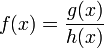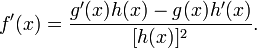Pathways To Learning... Since 2005 Hong Kong Registered School 566985

Menu

# Q - Q quotient rule - Mathematics Dictionary

A   B   C   D   E   F   G   H   I   J   K   L   M   N   O   P   Q   R   S   T   U   V   W   X   Y   Z

Q Usually printed in bold type, or written in Blackboard Bold to denote the set of all rational numbers.

Q1 The first quartile, that is, the lower quartile of a sample.

Q2 The second quartile, that is the median of a sample.

Q3 The third quartile, that is, the upper quartile of a sample.

QED Abbreviation for the Latin phrase "quod erat demonstrandum", meaning "which was to be demonstrated". Used to indicate the end of a proof, occasionally denoted by a square.

QEF Abbreviation for the Latin phrase "quod erat faciendum", meaning "which was to be done". Occasionally used to indicate the completion of a (usually geometric) construction.

QEI Abbreviation for the Latin phrase "quod erat inveniendum", meaning "which was to be found". Occasionally used to indicate the completion of a calculation.

quadrangle A 2D shape with 4 sides, also called a quadrilateral or a tetragon, although quadrilateral is the preferred term these days.

quadrangular prism: A prism with a quadrangular (quadrilateral) base.

quadrant One of the four regions in a plane divided by the axes in the Cartesian coordinate system.

quadrantal angle: Any angles which are multiples of right angles.

quadrantal spherical triangle: A triangle in spherical geometry where at least one side subtends an angle of a right angle from the centre. (centre of the sphere in forming the spherical coordinates, which may have to be deduced by backformation if the geometry arose otherwise)

quadratic That (expression, equation, function, term) of (maximum) degree 2. A quadratic polynomial thus contain a highest degree of 2.

quadratic formula: A formula derived from applying the method of completing the square to the general quadratic equation where the coefficients are unknown, that finds the roots of a quadratic equation. For an equation of the form ax2 + bx + c = 0, the formula isquadrature: The method of finding areas with the historical concept of equating any shape with a square of the same size.

quadric: A (hyper-)surface described by a single algebraic equation of order 2. (In n-dimensional space, the quadratic equation may consist of all n variables, and describes an (n-1)-dimensional hyper-surface.)

quadrilateral A 2D shape with 4 sides, also called a quadrangle or a tetragon, although quadrilateral is the preferred term these days.

quadruple: (To make to be) four-fold

quantiles: The boundary values when dividing a set of ordered data into parts of equal frequency. The values are known as percentiles for 100 parts, deciles for 10 parts, quartiles for 4 parts and the special class="d-title" names of the minimum, median and maximum for 2 parts.

quantum mechanics: A branch of physiics dealing with particles on the microscopic scale, it treats physical quantities such as momentum and energy as capable of only taking discrete values.

quartic That (expression, equation, function, term) of (maximum) degree 4. A quartic polynomialthus contain a highest degree of 4.

quartic polynomial: A 4th degree polynomial, that is, a polynomial of maximum degree of 4.

quartile 1. One of 4 parts that a sample into which, arranged in order, is divided.

2. A value below which a whole number of quarters of the data fall.

quartile deviation Also known as the semi-interquartile range, that is, half of the interquartile range.

quintal system: A base 5 number system.

quintic That (expression, equation, function, term) of (maximum) degree 5. A quintic polynomial thus contain a highest degree of 5.

quintic polynomial: A 5th degree polynomial. The lowest order polynomial, for which there is no general formula for finding the roots.

quota sample: A method of non-random sampling where the population is divided into groups and each group is given a quota - a proportion with which sample units from this group should form the sample. The fact that actions have to be taken in order to ensure that the proportions are right means that such samples may be biased.

quotient The result of a division, much as a product is the result of a multiplication.

quotient rule A rule for differentiation of a quotient of functions that is derived from applying chain rule and product rule to the general expression uv-1.A   B   C   D   E   F   G   H   I   J   K   L   M   N   O   P   Q   R   S   T   U   V   W   X   Y   Z